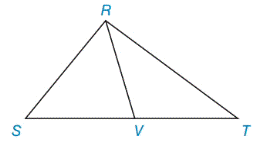Chapter 8.1, Problem 30E### Elementary Geometry for College St...

6th Edition
Daniel C. Alexander + 1 other
ISBN: 9781285195698

#### Solutions

Chapter
Section### Elementary Geometry for College St...

6th Edition
Daniel C. Alexander + 1 other
ISBN: 9781285195698
Textbook Problem
1 views

# The following problem is based on this theorem: “A median of a triangle separates it into two triangles of equal area.”In the figure, △ R S T has median R V ¯ .a) Explain why A R S V = A R V T .b) If A R S T = 40.8  cm 2 , find A R S V .To determine

a)

To explain:

ARSV=ARVT. If ΔRST has median RV¯

Explanation

Given,

In figure, ΔRST has median RV¯

Here, RV¯ separates the triangles RST into two triangles ΔRSV and ΔRVT.

Since, RV¯ is the median of ST¯

Let b be the length of base of ΔRST

(i.e.) ST¯=b and

Then, SV¯=b2 and VT¯=b2

(i.e.) SV¯=VT¯=b2

In both triangle of ΔRSV and ΔRVT, height is same

To determine

b)

To find:

ARSV if ARST=40.8 cm2.

### Still sussing out bartleby?

Check out a sample textbook solution.

See a sample solution

#### The Solution to Your Study Problems

Bartleby provides explanations to thousands of textbook problems written by our experts, many with advanced degrees!

Get Started